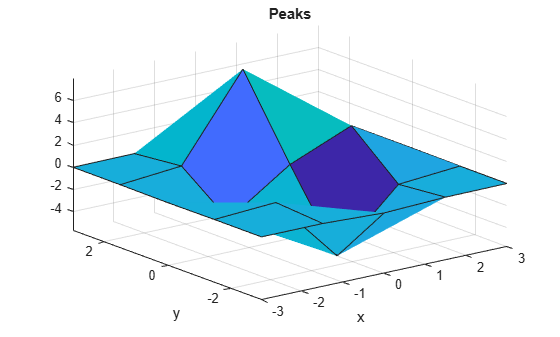# peaks

Example function of two variables

## Syntax

```Z = peaks; Z = peaks(n); Z = peaks(V); Z = peaks(X,Y); peaks(...) [X,Y,Z] = peaks(...); ```

## Description

`peaks` is a function of two variables, obtained by translating and scaling Gaussian distributions, which is useful for demonstrating `mesh`, `surf`, `pcolor`, `contour`, and so on.

`Z = peaks;` returns a 49-by-49 matrix.

`Z = peaks(n);` returns an `n`-by-`n` matrix.

`Z = peaks(V);` returns an `n`-by-`n` matrix, where ```n = length(V)```.

`Z = peaks(X,Y);` evaluates `peaks` at the given `X` and `Y` (which must be the same size) and returns a matrix the same size.

`peaks(...)` (with no output argument) plots the peaks function with `surf`. Use any of the input argument combinations in the previous syntaxes.

`[X,Y,Z] = peaks(...);` returns two additional matrices, `X` and `Y`, for parametric plots, for example, `surf(X,Y,Z,del2(Z))`. If not given as input, the underlying matrices `X` and `Y` are

```[X,Y] = meshgrid(V,V) ```

where `V` is a given vector, or `V` is a vector of length `n` with elements equally spaced from -3 to 3. If no input argument is given, the default `n` is 49.

## Examples

collapse all

Create a 5-by-5 matrix of peaks and display the surface.

```figure peaks(5);```
``` z = 3*(1-x).^2.*exp(-(x.^2) - (y+1).^2) ... - 10*(x/5 - x.^3 - y.^5).*exp(-x.^2-y.^2) ... - 1/3*exp(-(x+1).^2 - y.^2) ```